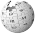## FANDOM

17,149 Pages

A square inch (plural: square inches) is a unit of area, equal to the area of a square with sides of one inch. The following symbols are used to denote square inches:

• square in
• sq inches, sq inch, sq in
• inches/-2, inch/-2, in/-2
• inches^2, inch^2, in^2
• inches², inch², in²

The square inch is a common unit of measurement in the United States and the United Kingdom.

## Equivalence with other units of areaEdit

1 square inch (assuming an international inch) is equal to: (the overbars indicate repeating decimals)

• 0.006 94 square feet (1 square foot is equal to 144 square inches)
• 0.000 771 604 9382 square yards (1 square yard is equal to 1,296 square inches)
• 6.4516 square centimetres (1 square centimetre is equal to 0.155 000 310… square inches)
• 0.000 645 16 square metres (1 square metre is equal to 1,550.003 100… square inches)

## References / sourcesEdit

Based on the wikipedia article - to define a common term used in articles.

{{{unit name}}}
Unit sign {{{sign}}}
Measure [[{{{measure}}}]]
Base Unit [[{{{base}}}]]
Multiple of Base 10{{{multiple}}}
System {{{system}}}
Common usage {{{usage}}}
Examples
{{{examples}}}
Conversion
SI {{{SI conversion}}}
Imperial {{{imperial conversion}}}This page uses some content from Wikipedia. The original article was at Square inch. The list of authors can be seen in the page history. As with Tractor & Construction Plant Wiki, the text of Wikipedia is available under the Creative Commons by Attribution License and/or GNU Free Documentation License. Please check page history for when the original article was copied to Wikia